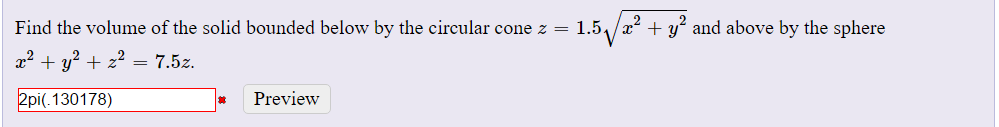Find the volume of the solid bounded below by the circular cone and above the sphereI'm asked to find the volume of the solid bounded below by the circular cone $$z= 1.5\sqrt(x^2+y^2)$$and above the sphere $$x^2+y^2+z^2=7.5z.$$

I tried to solve this using spherical coordinates, which gave me the bounds $$0 <= \rho <= 7.5 cos(\phi)$$ $$0 <= \phi <= arccot(1.5)$$ $$0<= \theta <= 2\pi$$ Are my bounds correct?

The spherical coordinates: $$x= \rho \cos(\theta)\sin(\phi)$$ $$y= \rho \sin(\theta)\sin(\phi)$$ $$z= \rho \cos(\phi)$$ We have $$x^2+y^2+z^2\leq7.5z \Rightarrow 0 \leq \rho \leq 7.5 \cos(\phi)$$ $$1.5\sqrt{x^2+y^2}=z \Rightarrow 1.5\sqrt{\rho^2 \sin^2(\phi)}=\rho \cos(\phi)\Leftrightarrow \operatorname{ctg}(\phi)=1.5$$ We have to find volume of the solid BELOW $z=1.5\sqrt{x^2+y^2}$ and ABOVE $x^2+y^2+z^2\leq7.5z$, so for azimuthal angle $\phi$ we have $$\operatorname{arcctg}(1.5) \leq \phi \leq \frac{\pi}{2},$$ and $$0 \leq \theta \leq 2 \pi.$$
P.S In case we have to find volume of the solid ABOVE $z=1.5\sqrt{x^2+y^2}$ and BELOW $x^2+y^2+z^2\leq7.5z$ , then $0 \leq \phi \leq \operatorname{arcctg}(1.5)$.